# Fisseha Berhane, PhD

#### Data Scientist443-970-2353[email protected] CV Resume#### Using MongoDB with R and Python¶

MongoDB is a NoSQL database program which uses JSON-like documents with schemas. It is free and open-source cross-platform database. MongoDB, top NoSQL database engine in use today, could be a good data storage alternative when analysing large volume data.

To use MongoDB with R and Python, first, we have to download and install MongoDB

Next, start MongoDB. We can start MongoDB like so:

In [ ]:
mongod


For R, we can use the mongolite package which is a fast and simple MongoDB client for R. For Python, on the other hand, we can use the PyMongo Python driver which is a Python distribution containing tools for working with MongoDB.

###### R¶
In [ ]:
install.packages("mongolite",dependencies =TRUE,repos='http://cran.us.r-project.org')

###### Python¶

We can use pip or easy_install as below:

In [ ]:
pip install pymongo

In [ ]:
easy_install pymongo


Or if we are using Anaconda distribution, we can install it with conda:

In [ ]:
conda install -c anaconda pymongo=3.3.0

##### Inserting data¶

Let's insert the crimes data from data.gov to MongoDB and query it using both R and Python. The dataset reflects reported incidents of crime (with the exception of murders where data exists for each victim) that occurred in the City of Chicago since 2001.

In :
library(ggplot2)
library(dplyr)
library(maps)
library(ggmap)
library(mongolite)
library(lubridate)
library(gridExtra)

In :
crimes=data.table::fread("Crimes_2001_to_present.csv")

Read 6261148 rows and 22 (of 22) columns from 1.371 GB file in 00:00:51

In :
cat("The crimes data has ",nrow(crimes)," observation and ",ncol(crimes)," columns")

The crimes data has  6261148  observation and  22  columns
In :
names(crimes)

1. 'ID'
2. 'Case Number'
3. 'Date'
4. 'Block'
5. 'IUCR'
6. 'Primary Type'
7. 'Description'
8. 'Location Description'
9. 'Arrest'
10. 'Domestic'
11. 'Beat'
12. 'District'
13. 'Ward'
14. 'Community Area'
15. 'FBI Code'
16. 'X Coordinate'
17. 'Y Coordinate'
18. 'Year'
19. 'Updated On'
20. 'Latitude'
21. 'Longitude'
22. 'Location'

Let's remove spaces in the column names to avoid any problems related with that.

In :
names(crimes) = gsub(" ","",names(crimes))
names(crimes)

1. 'ID'
2. 'CaseNumber'
3. 'Date'
4. 'Block'
5. 'IUCR'
6. 'PrimaryType'
7. 'Description'
8. 'LocationDescription'
9. 'Arrest'
10. 'Domestic'
11. 'Beat'
12. 'District'
13. 'Ward'
14. 'CommunityArea'
15. 'FBICode'
16. 'XCoordinate'
17. 'YCoordinate'
18. 'Year'
19. 'UpdatedOn'
20. 'Latitude'
21. 'Longitude'
22. 'Location'

With R, we can use the insert function from the mongolite package to insert rows to a collection in MongoDB. In Python, we can use the insert_one() method and the insert_many() method to add documents to a collection in MongoDB. In the next steps, I will show the commands that perform similar tasks with both R and Python. The Python codes are commented out.

Let's create a database called Chicago and call the collection crimes.

In :
my_collection = mongo(collection = "crimes", db = "Chicago") # create connection, database and collection
my_collection$insert(crimes) # insert data # from pymongo import MongoClient # Create a MongoClient to the running mongod instance. # client = MongoClient() # Connect on the default host and port # db = client.Chicago # database # my_collection=db.crimes # collection # my_collection.insert_one(crimes) # insert crimes data  Let's check if we have inserted the "crimes" data. In : my_collection$count()

# my_collection.count()

6261148

We see that the count() function above and nrow() give the same number of records

###### Performing a query and retrieving data¶

First, let's look what the data looks like by displaying one record:

In :
my_collection$iterate()$one()

# In Python, we use find_one() to get the first document in the collection
# my_collection.find_one()

$ID 1454164$CaseNumber
'G185744'
$Date '04/01/2001 06:00:00 PM'$Block
'049XX N MENARD AV'
$IUCR '0910'$PrimaryType
'MOTOR VEHICLE THEFT'
$Description 'AUTOMOBILE'$LocationDescription
'STREET'
$Arrest 'false'$Domestic
'false'
$Beat 1622$District
16
$FBICode '07'$XCoordinate
1136545
$YCoordinate 1932203$Year
2001
$UpdatedOn '08/17/2015 03:03:40 PM'$Latitude
41.970129962
$Longitude -87.773302309$Location
'(41.970129962, -87.773302309)'

How many distinct "Primary Type" do we have?

In :
length(my_collection$distinct("PrimaryType")) # len(my_collection.distinct("PrimaryType"))  35 As shown above, there are 35 different crime primary types in the database. We will see the patterns of the most common crime types below. From the$iterate()one() command above, we have seen that one of the columns is Domestic, which shows whether the crime is domestic or not. Now, let's see how many domestic assualts there are in the collection.

In :
my_collection$count('{"PrimaryType" : "ASSAULT", "Domestic" : "true" }') # my_collection.count({"PrimaryType" : "ASSAULT", "Domestic" : "true" })  82470 We can check with dplyr, like so: In : crimes%>%filter(PrimaryType=="ASSAULT",Domestic=="true")%>%nrow()  82470 To get the filtered data: In : query1= my_collection$find('{"PrimaryType" : "ASSAULT", "Domestic" : "true" }')
# query1=my_collection.find({"Primary Type" : "ASSAULT", "Domestic" : "true" }) #  find() returns a Cursor instance, which allows us to iterate over all matching documents

 Imported 82470 records. Simplifying into dataframe...


We can also retrieve only the columns of interest:

In :
query2= my_collection$find('{"PrimaryType" : "ASSAULT", "Domestic" : "true" }', fields = '{"_id":0, "PrimaryType":1, "Domestic":1}') #query2= my_collection.find('{"PrimaryType" : "ASSAULT", "Domestic" : "true" }','{"_id":0, "PrimaryType":1, "Domestic":1}')   Imported 82470 records. Simplifying into dataframe...  In : ncol(query1) # with all the columns ncol(query2) # only the selected columns  22 2 In : head(query2,4)  PrimaryTypeDomestic ASSAULTtrue ASSAULTtrue ASSAULTtrue ASSAULTtrue How do we do the above two queries with dplyr: In : query1=crimes%>%filter(PrimaryType=="ASSAULT", Domestic=="true" ) query2=crimes%>%filter(PrimaryType=="ASSAULT", Domestic=="true" )%>%select(PrimaryType,Domestic)  In : ncol(query1) ncol(query2)  22 2 Where do most crimes take pace? In : my_collection$aggregate('[{"$group":{"_id":"$LocationDescription", "Count": {"$sum":1}}}]')%>%na.omit()%>% arrange(desc(Count))%>%head(10)%>% ggplot(aes(x=reorder(_id,Count),y=Count))+ geom_bar(stat="identity",color='skyblue',fill='#b35900')+geom_text(aes(label = Count), color = "blue") +coord_flip()+xlab("Location Description")   Imported 174 records. Simplifying into dataframe...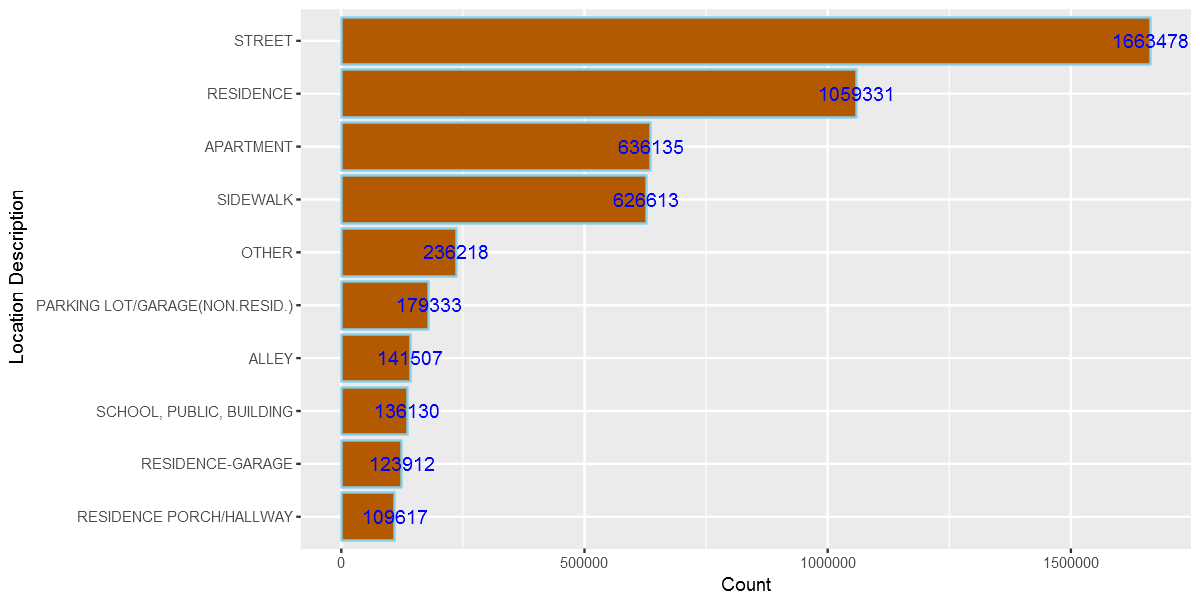If loading the entire dataset we are working with does not slow down our analysis, we can use data.table or dplyr but when dealing with big data, using MongoDB can give us performance boost as the whole data will not be loaded into mememory. We can reproduce the above plot without using MongoDB, like so: In : crimes%>%group_by(LocationDescription)%>%summarise(Total=n())%>% arrange(desc(Total))%>%head(10)%>% ggplot(aes(x=reorder(LocationDescription,Total),y=Total))+ geom_bar(stat="identity",color='skyblue',fill='#b35900')+geom_text(aes(label = Total), color = "blue") +coord_flip()+xlab("Location Description")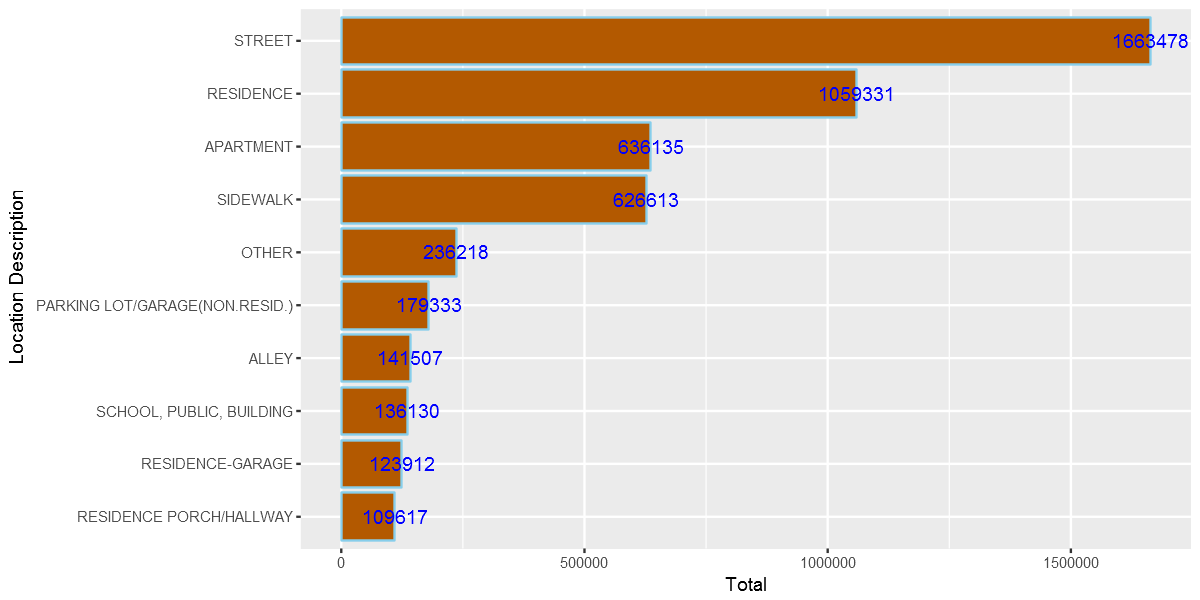What if we want to query all records for certain columns only? This helps us to load only the columns we want and to save memory for our analysis. In : query3= my_collection$find('{}', fields = '{"_id":0, "Latitude":1, "Longitude":1,"Year":1}')
# query3= my_collection.find('{}','{"_id":0, "Latitude":1, "Longitude":1}')

 Imported 6261148 records. Simplifying into dataframe...

##### Let's explore domestic crimes¶

We can explore any patterns of domestic crimes. For example, are they common in certain days/hours/months?

In :
domestic=my_collection$find('{"Domestic":"true"}', fields = '{"_id":0, "Domestic":1,"Date":1}') domestic$Date= mdy_hms(domestic$Date) domestic$Weekday = weekdays(domestic$Date) domestic$Hour = hour(domestic$Date) domestic$month = month(domestic$Date,label=TRUE)   Imported 806839 records. Simplifying into dataframe...  In : WeekdayCounts = as.data.frame(table(domestic$Weekday))
WeekdayCounts$Var1 = factor(WeekdayCounts$Var1, ordered=TRUE, levels=c("Sunday", "Monday", "Tuesday", "Wednesday", "Thursday", "Friday","Saturday"))
ggplot(WeekdayCounts, aes(x=Var1, y=Freq)) + geom_line(aes(group=1),size=2,color="red") + xlab("Day of the Week") + ylab("Total Domestic Crimes")+
ggtitle("Domestic Crimes in the City of Chicago Since 2001")+
theme(axis.title.x=element_blank(),axis.text.y = element_text(color="blue",size=11,angle=0,hjust=1,vjust=0),
axis.text.x = element_text(color="blue",size=11,angle=0,hjust=.5,vjust=.5),
axis.title.y = element_text(size=14),
plot.title=element_text(size=16,color="purple",hjust=0.5))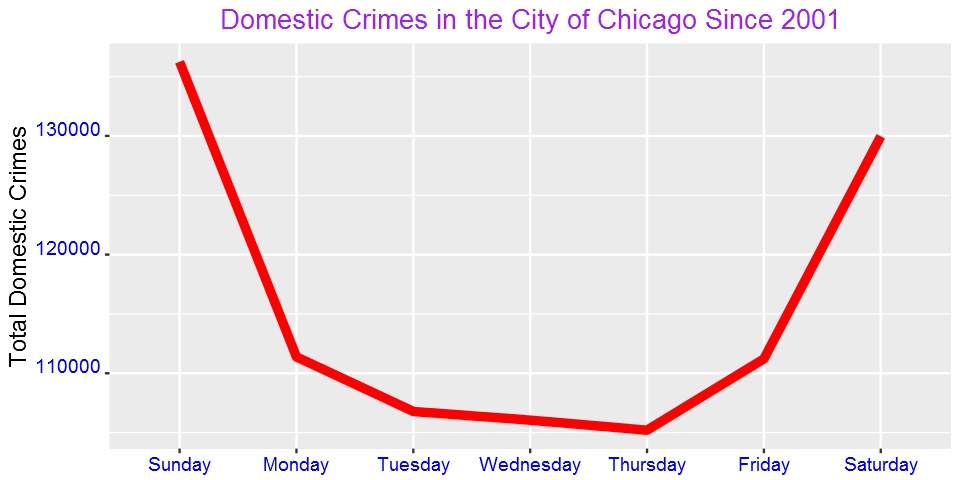Domestic crimes are common over the weekend than in weekdays? What could be the reason?

We can also see the pattern for each day by hour

In :
DayHourCounts = as.data.frame(table(domestic$Weekday, domestic$Hour))
DayHourCounts$Hour = as.numeric(as.character(DayHourCounts$Var2))
ggplot(DayHourCounts, aes(x=Hour, y=Freq)) + geom_line(aes(group=Var1, color=Var1), size=1.4)+ylab("Count")+
ylab("Total Domestic Crimes")+ggtitle("Domestic Crimes in the City of Chicago Since 2001")+
theme(axis.title.x=element_text(size=14),axis.text.y = element_text(color="blue",size=11,angle=0,hjust=1,vjust=0),
axis.text.x = element_text(color="blue",size=11,angle=0,hjust=.5,vjust=.5),
axis.title.y = element_text(size=14),
legend.title=element_blank(),
plot.title=element_text(size=16,color="purple",hjust=0.5))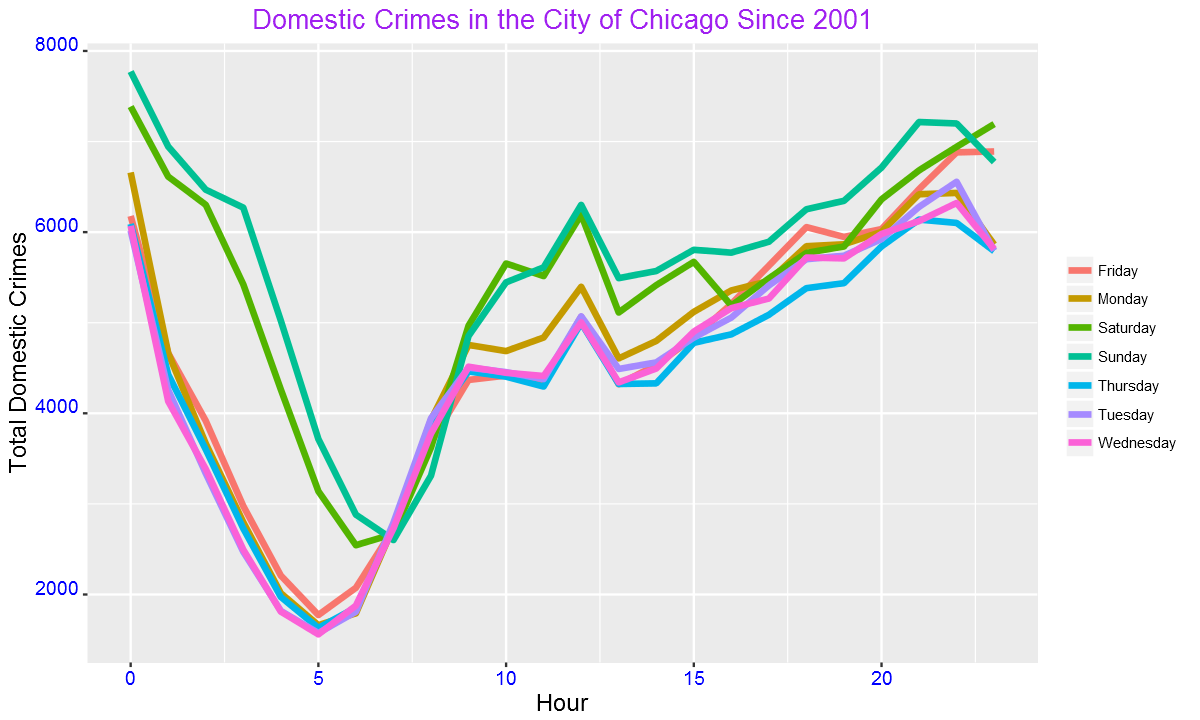The crimes peak mainly around mid-night. We can also use one color for weekdays and another color for weekend as shown below.

In :
DayHourCounts$Type = ifelse((DayHourCounts$Var1 == "Sunday") | (DayHourCounts$Var1 == "Saturday"), "Weekend", "Weekday") ggplot(DayHourCounts, aes(x=Hour, y=Freq)) + geom_line(aes(group=Var1, color=Type), size=2, alpha=0.5) + ylab("Total Domestic Crimes")+ggtitle("Domestic Crimes in the City of Chicago Since 2001")+ theme(axis.title.x=element_text(size=14),axis.text.y = element_text(color="blue",size=11,angle=0,hjust=1,vjust=0), axis.text.x = element_text(color="blue",size=11,angle=0,hjust=.5,vjust=.5), axis.title.y = element_text(size=14), legend.title=element_blank(), plot.title=element_text(size=16,color="purple",hjust=0.5))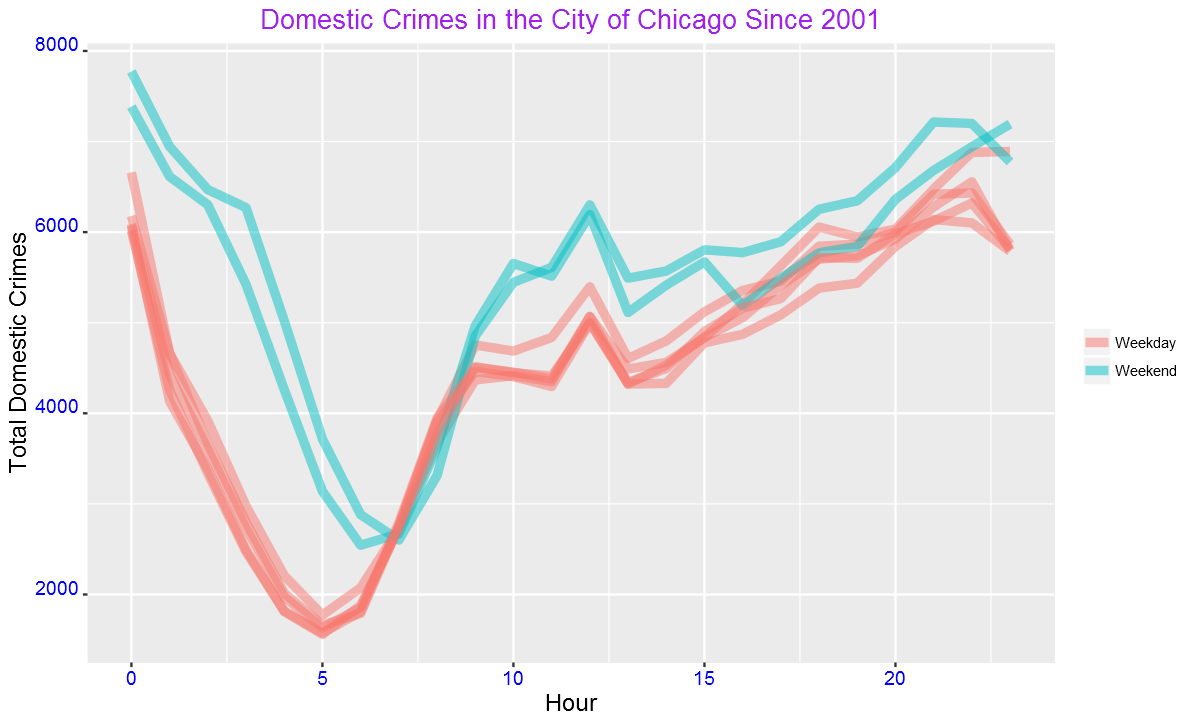The difference between weekend and weekdays are more clear from this figure than from the previous plot. We can also see the above pattern from a heatmap. In : DayHourCounts$Var1 = factor(DayHourCounts$Var1, ordered=TRUE, levels=c("Monday", "Tuesday", "Wednesday", "Thursday", "Friday", "Saturday", "Sunday")) ggplot(DayHourCounts, aes(x = Hour, y = Var1)) + geom_tile(aes(fill = Freq)) + scale_fill_gradient(name="Total MV Thefts", low="white", high="red") + ggtitle("Domestic Crimes in the City of Chicago Since 2001")+theme(axis.title.y = element_blank())+ylab("")+ theme(axis.title.x=element_text(size=14),axis.text.y = element_text(size=13), axis.text.x = element_text(size=13), axis.title.y = element_text(size=14), legend.title=element_blank(), plot.title=element_text(size=16,color="purple",hjust=0.5))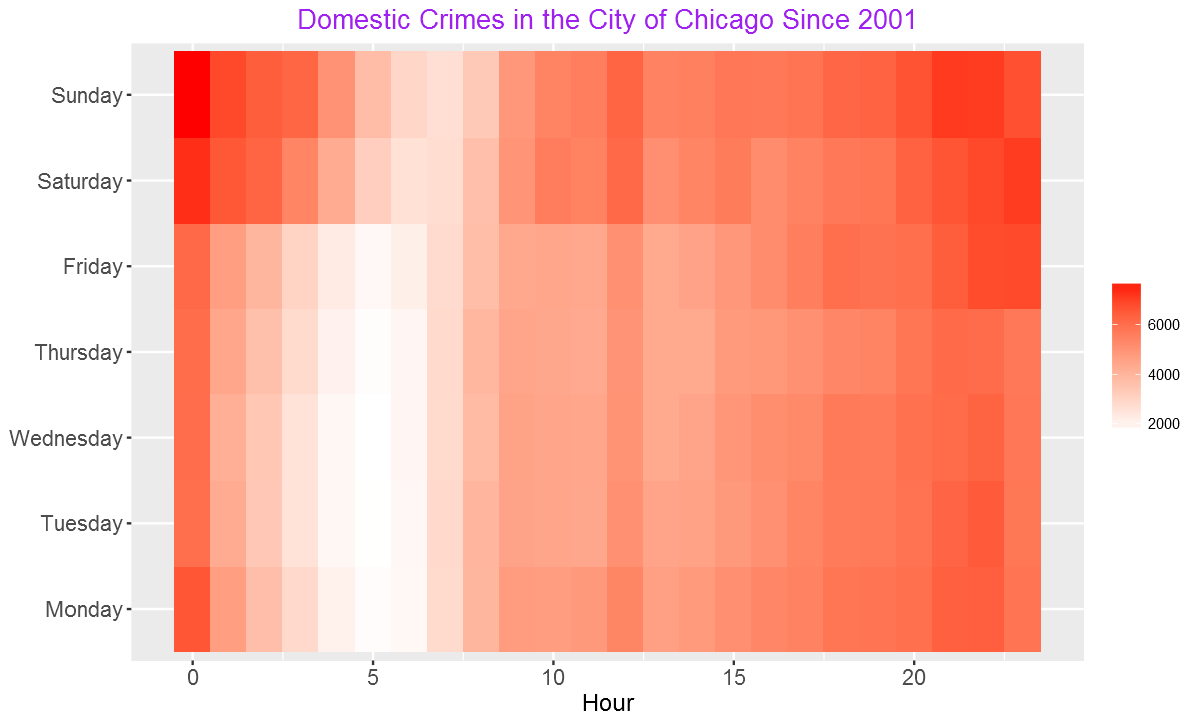From the heatmap, we can see more crimes over weekends and at night. In : monthCounts = as.data.frame(table(domestic$month))
ggplot(monthCounts, aes(x=Var1, y=Freq)) + geom_line(aes(group=1),size=2,color="red") + xlab("Day of the Week") + ylab("Total Domestic Crimes")+
ggtitle("Domestic Crimes in the City of Chicago Since 2001")+
theme(axis.title.x=element_blank(),axis.text.y = element_text(color="blue",size=11,angle=0,hjust=1,vjust=0),
axis.text.x = element_text(color="blue",size=11,angle=0,hjust=.5,vjust=.5),
axis.title.y = element_text(size=14),
plot.title=element_text(size=16,color="purple",hjust=0.5))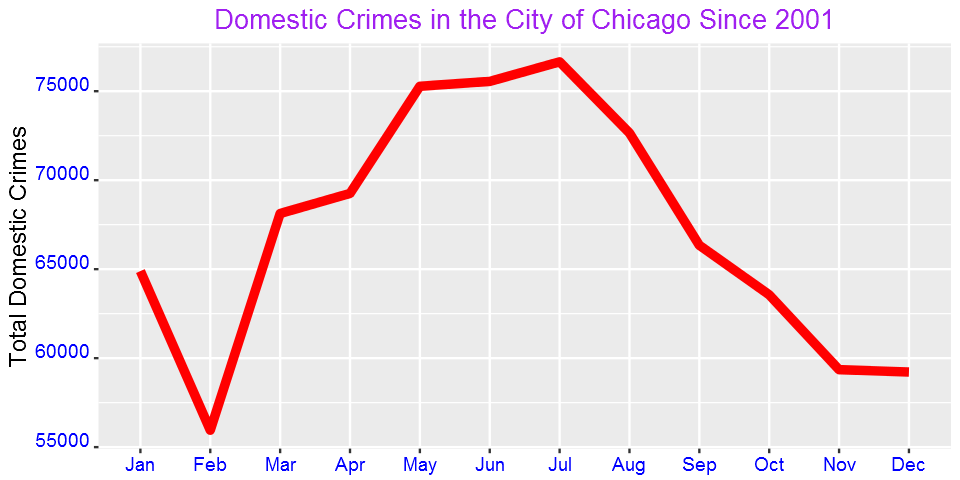Is domestic crime associated with tempreature? Domestic crimes tremendoulsy increases during the warm months.

Now, let's see the pattern of other crime types. Since there are 35 primary types, we cannot see all of them in this post. Let's focus on four of the most common ones.

In :
crimes=my_collection$find('{}', fields = '{"_id":0, "PrimaryType":1,"Year":1}') crimes%>%group_by(PrimaryType)%>%summarize(Count=n())%>%arrange(desc(Count))%>%head(4)   Imported 6261148 records. Simplifying into dataframe...  PrimaryTypeCount THEFT 1301434 BATTERY 1142377 CRIMINAL DAMAGE 720143 NARCOTICS 687790 As shown in the table above, the most common crime type is theft followed by battery. Narcotics is fourth most common while criminal damage is the third most common crime type in the city of Chicago. Now, let's generate plots by day and hour. In : four_most_common=crimes%>%group_by(PrimaryType)%>%summarize(Count=n())%>%arrange(desc(Count))%>%head(4) four_most_common=four_most_common$PrimaryType

In :
crimes=my_collection$find('{}', fields = '{"_id":0, "PrimaryType":1,"Date":1}') crimes=filter(crimes,PrimaryType %in%four_most_common) crimes$Date= mdy_hms(crimes$Date) crimes$Weekday = weekdays(crimes$Date) crimes$Hour = hour(crimes$Date) crimes$month=month(crimes$Date,label = TRUE)   Imported 6261148 records. Simplifying into dataframe...  In : g = function(data){ WeekdayCounts = as.data.frame(table(data$Weekday))
WeekdayCounts$Var1 = factor(WeekdayCounts$Var1, ordered=TRUE, levels=c("Sunday", "Monday", "Tuesday", "Wednesday", "Thursday", "Friday","Saturday"))
ggplot(WeekdayCounts, aes(x=Var1, y=Freq)) + geom_line(aes(group=1),size=2,color="red") + xlab("Day of the Week") +
theme(axis.title.x=element_blank(),axis.text.y = element_text(color="blue",size=10,angle=0,hjust=1,vjust=0),
axis.text.x = element_text(color="blue",size=10,angle=0,hjust=.5,vjust=.5),
axis.title.y = element_text(size=11),
plot.title=element_text(size=12,color="purple",hjust=0.5))
}

In :
g1=g(filter(crimes,PrimaryType=="THEFT"))+ggtitle("Theft")+ylab("Total Count")
g2=g(filter(crimes,PrimaryType=="BATTERY"))+ggtitle("BATTERY")+ylab("Total Count")
g3=g(filter(crimes,PrimaryType=="CRIMINAL DAMAGE"))+ggtitle("CRIMINAL DAMAGE")+ylab("Total Count")
g4=g(filter(crimes,PrimaryType=="NARCOTICS"))+ggtitle("NARCOTICS")+ylab("Total Count")
grid.arrange(g1,g2,g3,g4,ncol=2)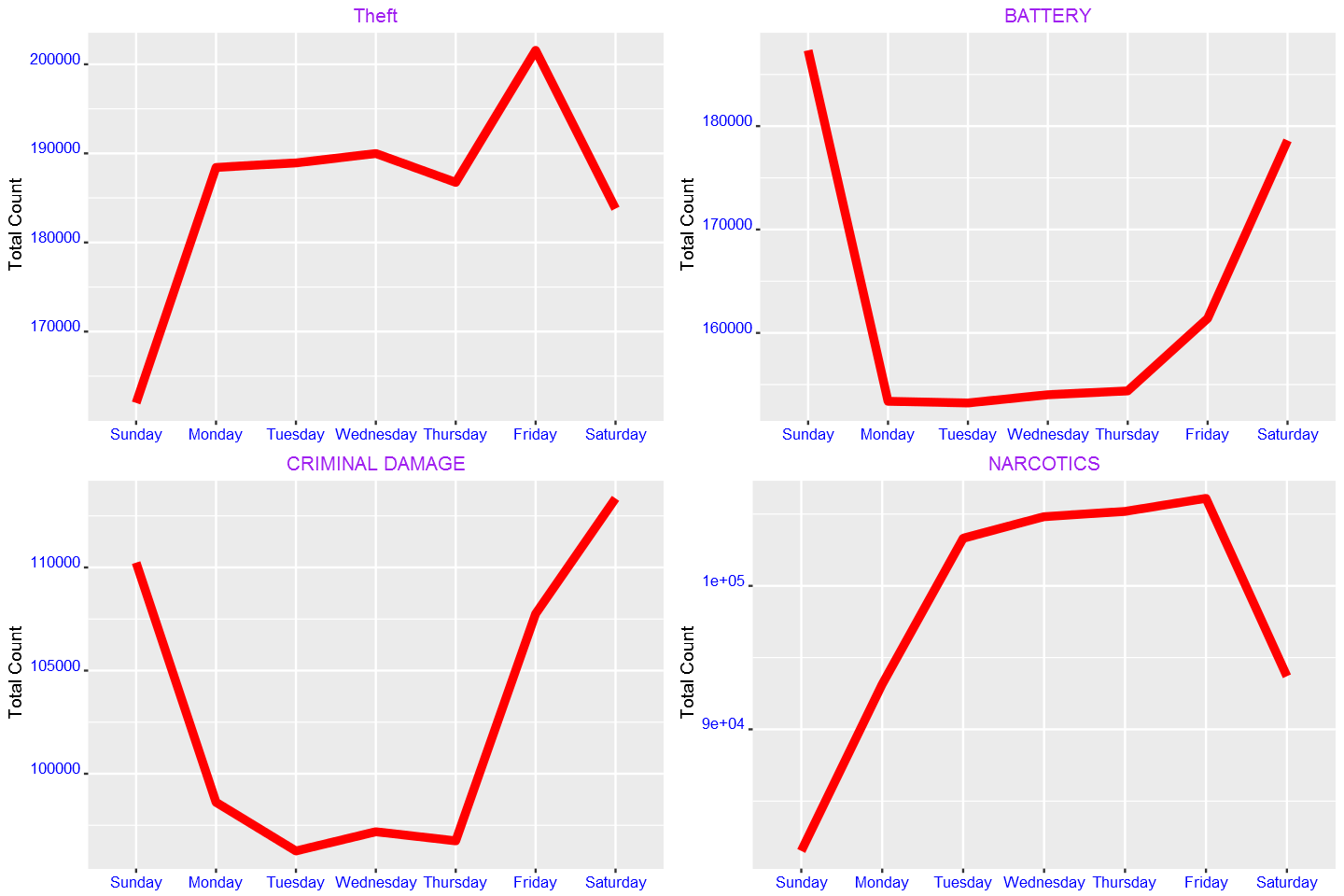. From the plots above, we see that theft is most common on Friday. Battery and criminal damage, on the other hand, are highest at weekend. We also observe that narcotics decreases over weekend.

We can also see the pattern of the above four crime types by hour:

In :
g=function(data){
DayHourCounts = as.data.frame(table(data$Weekday, data$Hour))
DayHourCounts$Hour = as.numeric(as.character(DayHourCounts$Var2))
ggplot(DayHourCounts, aes(x=Hour, y=Freq)) + geom_line(aes(group=Var1, color=Var1), size=1.4)+ylab("Count")+
theme(axis.title.x=element_text(size=14),axis.text.y = element_text(color="blue",size=11,angle=0,hjust=1,vjust=0),
axis.text.x = element_text(color="blue",size=11,angle=0,hjust=.5,vjust=.5),
axis.title.y = element_text(size=14),
legend.title=element_blank(),
plot.title=element_text(size=16,color="purple",hjust=0.5))
}

In :
g1=g(filter(crimes,PrimaryType=="THEFT"))+ggtitle("Theft")+ylab("Total Count")
g2=g(filter(crimes,PrimaryType=="BATTERY"))+ggtitle("BATTERY")+ylab("Total Count")
g3=g(filter(crimes,PrimaryType=="CRIMINAL DAMAGE"))+ggtitle("CRIMINAL DAMAGE")+ylab("Total Count")
g4=g(filter(crimes,PrimaryType=="NARCOTICS"))+ggtitle("NARCOTICS")+ylab("Total Count")
grid.arrange(g1,g2,g3,g4,ncol=2)